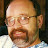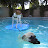## Inequality Story Problem 2 Airport Luggage – Tutor Algebra## Inequality Story Problem 2 Airport Luggage – Tutor Algebra

This math video tutors and gives instruction about solving inequalities. The instruction is a brief and to the point teaching lesson on how to learn algebra and manipulate variables.
Inequalities are solved similarly to equations, however, there are some very important differences.

algebra tutors,algebra,college,educational,equation,expression,for,ged,help,high,homework,how to,inequality,instruction,learn,lesson,linear,math,mathematics,online,practice,pre,property,school,simplify,solve,solving,teach,tutor,tutorial,tutoring,variable,video,with

algebratutors.org

1.Paul Bogdan says:
2.desertdoggz says: